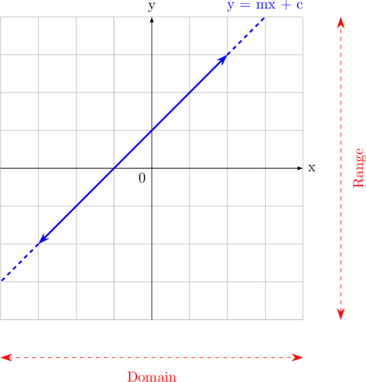# Domain and Range

## Linear Function

Linear function can be written as:

• $$f : x \rightarrow mx + c$$
• $$f(x) = mx + c$$
• $$y = mx + c$$

The value of x and y can be any number.

###### Domain and Range of Linear Function

Domain

$$x \in R$$

or can be written as

$$- \sim \: < x < +\sim$$

Range

$$y \in R$$

or can be written as

$$- \sim \: < y < +\sim$$

Linear function can be drawn in Cartesian diagram as a straight line like below: